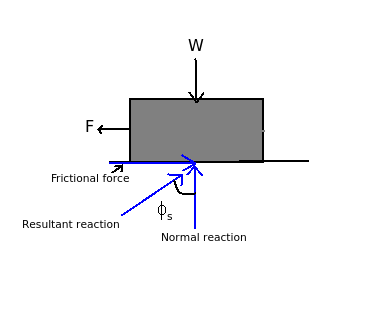# Formula to calculate angle of static friction

Q.  Which formula is used to calculate angle of static friction (Φs)?
- Published on 18 Sep 15

a. tan-1 μs
b. sin-1 μs
c. cos-1 μs
d. none of the above

#### Discussion

• Sravanthi   -Posted on 16 Dec 15
- In impending motion, the angle between normal reaction and resultant reaction is known as angle of static friction.- In the diagram shown,

tan Φs = (Frictional force) / (Normal reaction)

But coefficient of static friction (μs) = (Frictional force) / (Normal reaction)

tan Φs = (μs)

Therefore, Φs = tan-1 (μs)

## ➨ Post your comment / Share knowledgeEnter the code shown above:

(Note: If you cannot read the numbers in the above image, reload the page to generate a new one.)